A univariate time series describes a single variable that is measured at different points in time. The measurements are typically sequential, and spaced evenly in time. When analyzing such data, we want to develop a time dependent model that can explain past observations or forecast future trends. Usually, each measurement in a time series is influenced by the preceding data points. This implies the data is not independent, and standard regression models will not be suitable for the analysis.

I’ll begin this post by describing a special type of time series data, known as a stationary time series. In Section 2, we’ll discuss Wold’s theorem of decomposition, and the use of an autoregressive moving average (ARMA) model to forecast this type of data. The ARMA model consists of two halves — an auto regressive (AR) half that will be described in Section 3, and a moving average (MA) half that will be described in Section 4. In Section 6, we’ll describe a modification of the ARMA model which can be applied to nonstationary data. The final sections of this post will discuss how to properly implement these models in practice. Below is a table of contents to help you navigate these sections.

# Stationary Data

In time series analysis, we typically want to deal with stationary data. A time series is stationary if its mean, variance, and autocorrelations (correlations with prior deviations from the mean) are constant over time. A stationary series will have no upward or downward trends, will have consistent variations from the mean, and will have consistent short-term patterns.

Many time series do not meet above requirements. However, most time series can be made stationary by examining the differences of sequential measurements rather than the measurements themselves. We’ll discuss the details of this process in Section 6.

Selingkar Dakwat provides a number of stationary and nonstationary time series examples in his time series econometrics blog post. For your convenience, I’ve included his plots below. Note that the upward and downward trends in the latter plots make it easy to identify the data as nonstationary. This is not always the case, as it may be harder to recognize a time dependent variance by eye. In Section 7, we’ll introduce the autocorrelation function, which will provide a more quantitative measurement of the stationarity of a time series.

Figure 1: Stationary Time Series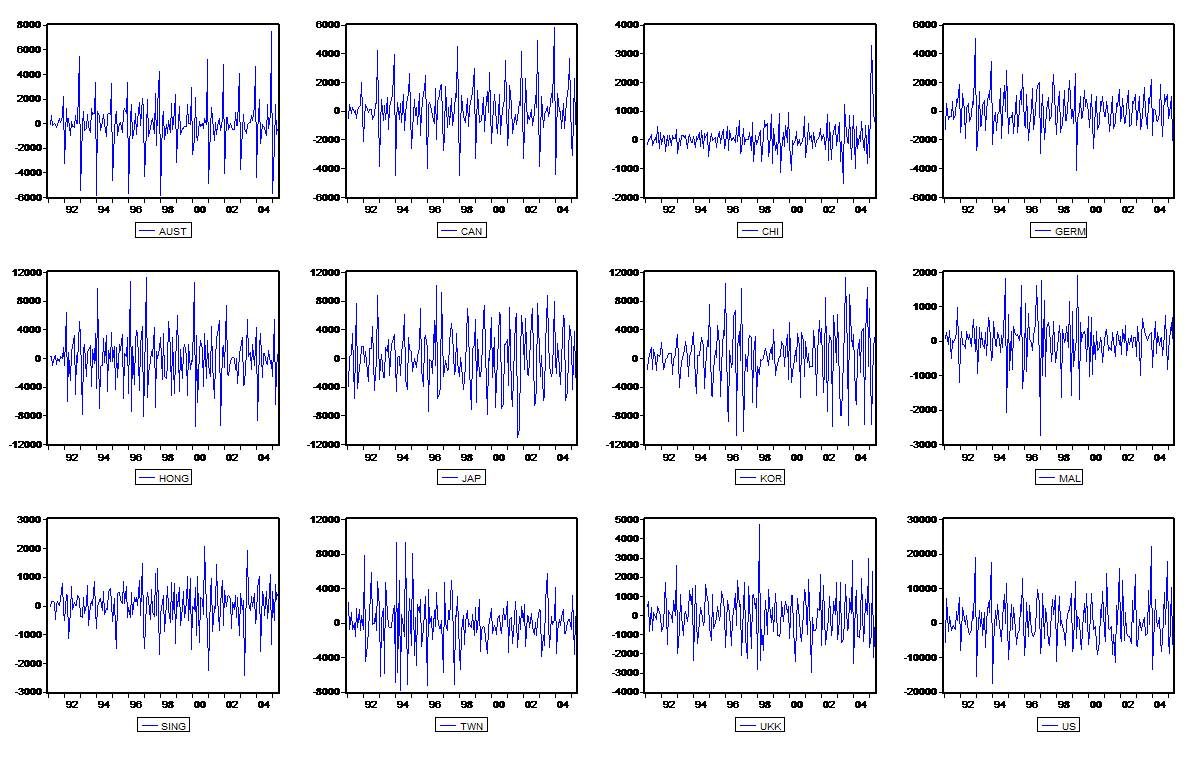Figure 2: Nonstationary Time Series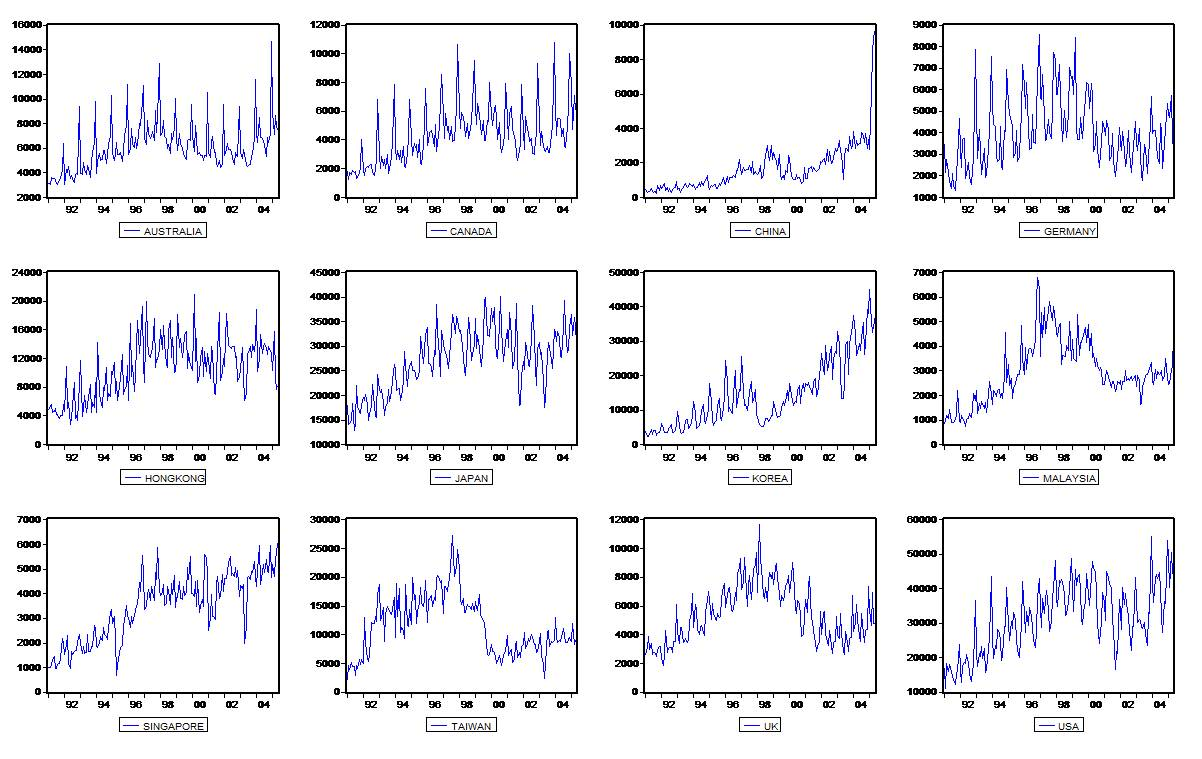# Wold’s Theorem of Decomposition

Wold’s theorem of decomposition states that any stationary process can be decomposed into a deterministic component and an indeterministic, stochastic component. Mathematically, this is written as

where $x_t$ is the value of the time series at time $t$, and $\epsilon_{t-j}$ term is the error of the model at the prior $x_{t-j}$ measurement. The first term on the right ($\eta_t$), is the deterministic component of the time series, and represents a perfectly predictable behavior based on past observations on $x_t$ alone. This resembles an autoregressive model, and will be discussed in Section 3. The second term on the right represents the indeterministic component of the series. It is a linear combination of past errors of the model due to random white noise, and represents a component of the time series that is impossible to predict perfectly. This resembles a moving average model, and will be discussed in section Section 4.

Wold’s theorem of decomposition motivates what is known as an autoregressive moving average (ARMA) model for stationary time series analysis. In the next two sections we’ll discuss the autoregressive (AR) and moving average (MA) terms separately, before detailing the full ARMA model in section Section 5.

# The Autoregressive Model

There are a few ways that we can model the time dependence of our data. First, we can use an autoregressive (AR) model which claims that the current observations are dependent on the previous observations. There are different orders of AR models, with the order denoting how many past observations (or “lags”) are used to predict the current observation. The order of an AR model is typically represented with with the letter $p$, and written as AR(p). For instance, a first order AR(1) model uses lag-1 data to predict the value at present time. The mathematical representation of this model would be

where $x_t$ is the observation at time t, $x_{(t-1)}$ is the lag-1 observation, $\phi$ are the model parameters, and $\epsilon_t$ is a stochastic error term representing random white noise. Notice that this is essentially a linear regression model, where we would optimize the model parameters by minimizing the sum of the squared error terms.

Similarly, an AR(2) model uses $x_{(t-1)}$ and $x_{(t-2)}$ to determine the value at $x_t$. Mathematically, this model would be represented by

From these examples, it’s easy to see that the general mathematical equation for an AR model of some order $p$ is given by:

# The Moving Average Model

We can also use a moving average (MA) model to describe our time series data. These models claim that the current observations are dependent on previous forecast errors. The order of an MA model is denoted with a $q$, and represents how many lag terms are included in the model. Mathematically, a first order MA(1) model is represented by

where we have distinguished the MA model parameters with $\theta$. In general, an MA(q) model of some order $q$ is written as

Note that the model parameters are defined with negative signs for historical reasons, and that some analysis programs may choose the opposite convention. It is also important to note that the moving average model is not equivalent to a linear regression model. Although the equations resemble each other, the $\epsilon$ terms are not directly observed, and must be computed on a measurement-by-measurement basis when the model is fitted to the data. Therefore, the predictors are not independent variables and the model parameters must be optimized using nonlinear methods, such as hill-climbing, rather than solving a system of equations as we would with linear regression.

A consequence of this model is that any shock to the time series will only propagate to $q$ observations in the future. In contrast, a shock to an AR model will directly affect every term in the series, since each observation is recursively dependent on the past observations. In this way, a shock to an AR model is propagated to all subsequent terms, whereas a shock to an MA model is propagated to a limited number of nearby terms.

# The ARMA Model

As mentioned in Section 2, stationary time series data can be modeled by a combination of the AR and MA models. This combined model is known as an autoregressive moving average (ARMA) model, and is represented mathematically by

where $C$ is a constant fit parameter, and $p$ and $q$ denote the orders of the AR and MA models, respectively. Note that is it possible for the ARMA model to reduce to a purely AR model if $p=0$, or a purely MA model if $q=0$.

# Differencing and the ARIMA Model

Recall our discussion of Wold’s decomposition theorem from Section 2. We stated that any stationary time series data can be approximated by an ARMA model. If the time series we’re working with isn’t stationary, we’ll need to take some extra steps to make it so. One way to accomplish this is to model the differences of each measurement in the dataset, rather than model the measurements themselves. This process helps to stabilize the mean of our model. An intuitive way to think about this is to imagine a time series which has a constant, upward slope. This series is not stationary, since the upward trend implies a time dependence in the mean of the data. However, since the slope is constant the difference between sequential points will be identical at all points on the line, and a time series of the differences will be flat and stationary.

An autoregressive integrate moving average (ARIMA) model incorporates this differencing operation, and is the most general class of models which can be applied to data that can be made stationary by differencing. In an ARIMA model, the data is differenced a number of times before the autoregressive and moving average terms are applied. The number of difference operations is typically denoted with the letter $d$. If $d = 0$, an ARIMA model reduces to the ARMA model. If $d = 1$, the time series is differenced one time, resulting in a new time series $X_t$, where

Likewise, if $d=2$ the time series being modeled is the difference of the differences:

After performing the differencing steps, the autoregressive and moving average terms are applied to the ARIMA model, such that

where $p$ is the number of autoregressive terms, $q$ is the number of moving average terms, and $d$ is the number of differences required to make the original time series stationary. Note that the only difference between the ARMA equation and the ARIMA equation is that the time series being modeled is the differenced series $X_t$, rather than the original series $x_t$. (If $d = 0$, the differenced series is equivalent to the original series).

# Determining the number of Differences

The hardest part of implementing an ARIMA(p,d,q) model is determining the appropriate number of differences, autoregressive terms, and moving average terms to include in the model.

Two plots, known as the autocorrelation function (ACF) and the partial autocorrelation function (PACF), are extremely useful when addressing these questions. An ACF plot is a bar graph of the coefficients of correlation between a time series and the lags of itself. If there is significant correlation between $x_t$ and $x_{t-1}$, as well as $x_{t-1}$ and $x_{t-2}$, then we should also find a correlation between $x_t$ and $x_{t-2}$. In this way, the correlation from lower lag terms will propagate to higher lag terms in the ACF plot. The PACF plot addresses this issue by plotting the partial correlation of coefficients between the time series and lags of itself, where the partial correlation between two variables is any correlation that is not explained by mutual relationships with lower order lag terms.

If the series exhibits any long-term trends, this will produce positive autocorrelations out to a high number of lags in the ACF plot. An example of this, taken from Duke University’s time series tutorial, is shown in Figure 3. In this case, the series must be differenced at least one more time before the data is stationary. Note that it is possible to “over-difference” a time series, resulting in an overly complicated model. To avoid this issue, you should only apply the minimum number of differences required to produce a flat mean in the series, and an ACF plot that decays to zero within a few lags.

Figure 3: Nonstationary ACF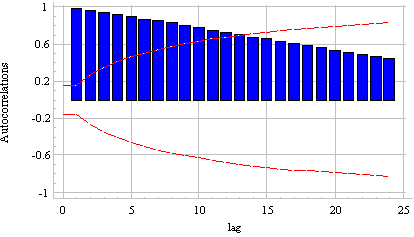In general, differencing introduces negative correlation to the series, driving the autocorrelation of the lag-1 term towards negative values. If the lag-1 autocorrelation becomes negative, the series does not need to be differenced further. If the lag-1 autocorrelation becomes less than -0.5, as it is in Figure 4, it is possible the series is over-differenced.

Figure 4: Over-differenced ACF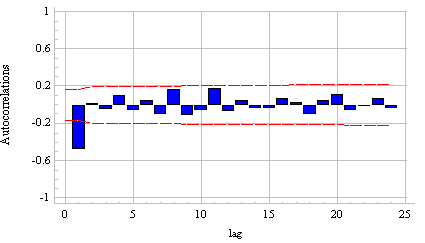If the lag-1 autocorrelation is close to 1.0, or if the sum of all autocorrelations is close to 1.0, the time series is said to have a “unit root.” This indicates that the series is nonstationary, and additional differencing operations should be applied. An example of this is shown in Figure 5. Similarly, if the lag-1 term of the PACF plot is close to 1.0, or if the sum of all of the partial autocorrelations is close to 1.0, the series has a unit root due to over-differencing. In this case, you should reduce the order of differencing in the model. A telltale sign of a unit root is erratic or unstable behavior in the long-term forecasts of your model. If such behavior is observed, you should reassess your ACF and PACF plots and reconsider the number of differencing operations that were included in your model.

Figure 5: Under-differenced ACF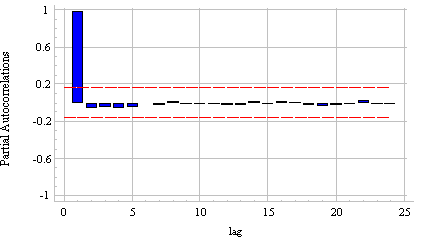The number of differencing operations we perform will determine whether or not we should include a constant term in our ARIMA model. The constant fit parameter, $C$, is almost always included in an undifferenced ARIMA(p,0,q) model, since the addition of the parameter allows for a non-zero, constant mean in the time series. In an ARIMA(p,1,q) model, the constant fit parameter allows for a non-zero average trend over the original time series. Therefore, if one difference operation has been applied the constant fit parameter should be included in the model if the original time series has a non-zero average trend. Similarly, in an ARIMA(p,2,q) model, the constant fit parameter allows for a non-zero average curvature in the original time series. It is rarely the case that we need to model a non-zero curvature in the original time series, so second order difference models generally do not include the constant term. Instead, data with a non-zero curvature (or with non-constant variance) can be addressed by transforming the initial series with a logarithm or square root operation.

Note: All plots in this section and the following section were taken from Duke University’s time series tutorial. For those looking for additional reading, I can’t recommend their website enough. It is by far the best tutorial I have come across.

# Determining the Number of AR terms

After applying the appropriate number of differences, any mild under-differencing or over-differencing can be addressed by adding AR or MA terms to the model. If the PACF displays a sharp cut off at a low number of lags, while the ACF decays slowly over a higher number of lags, we say the series displays an “AR signature.” An example of this is shown in Figures 6 and 7. These plots indicate that the autocorrelations of the differenced time series are best explained by adding additional AR terms to the model. In particular, the partial autocorrelation at lag-k (where k is indicating some order of the lag) is approximately equal to the AR(k) coefficient in an autoregressive model. Therefore, if the PACF falls below a statistical significance at lag-k, then you should add $k$ AR terms to your ARIMA model. Note that the cut-off of statistical significance is often ambiguous, with autocorrelations below 0.2 often being considered insignificant. In the case of Figure 7, the PACF indicates that we should add two AR terms to our model.

Figure 6: AR Signature in an ACF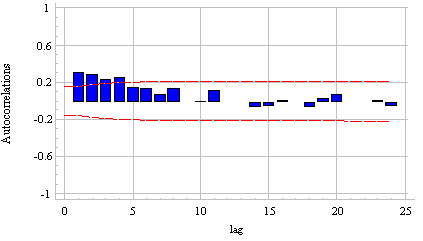Figure 7: AR Signature in a PACF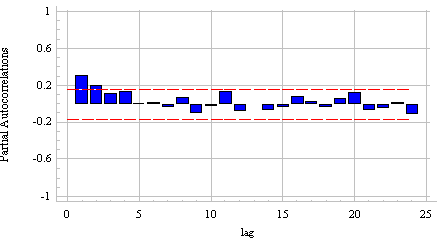An AR signature is often accompanied with a positive autocorrelation at lag-1, indicating a mild under-differencing in the time series. Therefore, it is helpful to think of AR terms as adding partial differences to the ARIMA model. This line of thinking is consistent with the unit roots discussed in the previous section, since an autocorrelation of approximately 1.0 at lag-1 indicates a significant under-differencing in the time series model. In fact, any autocorrelation pattern in a stationary time series can be removed by adding enough AR terms, even if doing so isn’t always the best approach. For instance, in the case of a unit root it is better to apply one full differencing operation at the beginning of the model, rather than apply multiple partial differencing operations via additional AR terms.

# Determining the number of MA terms

When determining the appropriate number of MA terms to add to your ARIMA model, the ACF plot plays an analogous role to the PACF plot in the previous section. That is, if the ACF plot displays a sharp cut off at a low number of lags, while the PACF decays slowly over a higher number of lags, the series displays an “MA signature.” In this case, if the ACF plot cuts off at lag-k, it indicates that the the autocorrelations of the differenced time series are best explained by adding exactly $k$ MA terms to our model. An MA signature is often accompanied with a negative autocorrelation at lag-1, indicating a mild over-differencing in the time series. As in the previous section, it is helpful to think of MA terms as partially canceling an order of differencing in our ARIMA model.

# Summary of Modeling Practices

The previous three sections can be summarized with the following list:

• If the ACF plot gradually falls off and extends to high lag terms, difference your model additional times

• If the lag-1 term in an ACF plot is close to 1.0, or if the sum of all ACF terms are close 1.0, then you should add an additional differencing operation

• If the lag-1 term in an PACF plot is close to 1.0, or if the sum of all ACF terms are close 1.0, then you should remove a differencing operation

• If the PACF cuts off sharply at lag-k, and the ACF falls off gradually, add k MA terms. Typically, this will be accompanied by a positive lag-1 term in the ACF plot.

• If the ACF cuts off sharply at lag-k, and the PACF falls off gradually, add k AR terms. Typically, this will be accompanied by a negative lag-1 term in the ACF plot.

As a final note, some time series will not conform to the rules above. These data sets will require a mixed model that includes both AR and MA terms. In this case, the AR and MA terms tend to work against each other, and it is easy for a model to spiral out of control by including incrementally more AR and MA terms. In general, if using a mixed ARIMA model you should favor models which use fewer terms. For instance, if an ARIMA(1,1,2) model fits the model well, it’s likely that the simpler ARIMA (0,1,1) model will work as well. This issue can usually be avoided by adding only one type of term in a step-by-step manner as described above.

# Evaluating an ARIMA model

As we’ve seen in this post, most time series analyses exploit the correlations between adjacent values to forecast future data. Since these models are trained on dependent data, common validation techniques such as Leave-One-Out cross validation are not applicable when evaluating the models’ performance. Instead, we can evaluate each model’s performance using the follow steps:

1) Fit the model using data up to a specific time, denoted by $x_T$.

2) Use the model to predict the next point in the time series, denoted as $\hat{x}_{T+1}$.

3) Compute the error of the prediction using $\epsilon_{T+1} = x_{T+1} - \hat{x}_{T+1}$, where $x_{T+1}$ is the true measurement at time $T+1$.

4) Repeat steps 1-3 for $T=m,\ldots,(n-1)$, where $m$ is the minimum number of measurements needed to evaluate the model, and $n$ is the total number of measurements in the time series. For instance, if a model implements lag-2 terms, $m=2$.

5) Compute the mean squared error using the results from step 4.

# Seasonal ARIMA models

Some time series have seasonal trends in the data. For instance, purchases of heavy coats would likely rise in winter, and fall in summer, every year. We can stationarize this type of data by taking the seasonal difference of the time series. That is, if the seasonal trends repeat every $S$ measurements, we can remove them by modeling the difference of $x_t - x_{t-S}$. In doing so, we force the model to predict future data based on the shape of the most recently observed seasonal pattern.

The implementation of a seasonal ARIMA model is identical to the standard ARIMA process, with that exception that the time series being modeled uses the seasonal differences of the data, rather than the differences of adjacent data. After differencing, seasonal AR and MA terms can be added to the model as we did in Sections 8 and 9. Note that each lag of a seasonal model uses data that is a multiple of the seasonal period. In other words, a lag-1 term would correspond to $x_t-x_{t-S}$, whereas a lag-2 term would correspond to $x_t-x_{t-2S}$.

Often, a purely seasonal model will not provide a satisfactory forecast of future data. In particular, since purely seasonal models forecast data using seasonal differences, sudden changes in the data are not reflected until an entire season has passed. To address this issue, we can add non-seasonal differences to the model. For instance, for data with a 12 month seasonal period, we may want to model the first difference of the season differences. That is, we may want to model:

This type of model is denoted as ARIMA(p,d,q)$\times$(P, D, Q)S, where $p$ is the non-seasonal AR order, $d$ is the non-seasonal differencing order, $q$ is the non-seasonal MA order, $P$ is the seasonal AR order, $D$ is the seasonal differencing order, $Q$ is seasonal MA order, and $S$ is number of measurements included in one seasonal pattern. Such a model can be broken down into four components. The short term, non-seasonal components are written:

while the long term, seasonal components are written:

# Closing remarks

In this post we learned the theory behind the ARIMA(p,d,q) model for forecasting time series data. Next time, I’ll implement the techniques we discussed in Sections 7-11 to analyze a time series in Python. At the moment I’m busy writing my dissertation and applying to jobs, so I expect the next post will be up in about two weeks.